矩阵乘法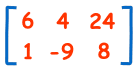（这矩阵有2行和3列）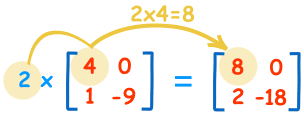2×4=8 2×0=0 2×1=2 2×-9=-18

矩阵与矩阵相乘"点积" 是把 对称的元素相乘，然后把结果加起来：

(1, 2, 3) • (7, 9, 11) = 1×7 + 2×9 + 3×11 = 58(1, 2, 3) • (8, 10, 12) = 1×8 + 2×10 + 3×12 = 64

(4, 5, 6) • (7, 9, 11) = 4×7 + 5×9 + 6×11 = 139

(4, 5, 6) • (8, 10, 12) = 4×8 + 5×10 + 6×12 = 154为什么要这样做？

例子：饼店卖三种派。

• 牛肉派卖￥3一个
• 鸡肉派卖￥4一个
• 素菜派卖￥2一个\$3×13 + \$4×8 + \$2×6 = \$83

(\$3, \$4, \$2) • (13, 8, 6) = ￥3×13 + ￥4×8 + ￥2×6 = ￥83

• 星期一的销售额是：牛肉派：￥3×13=￥39，鸡肉派：￥4×8=￥32，素菜派：￥2×6=￥12。总共是 ￥39 + ￥32 + ￥12 = ￥83
• 星期二的销售额是：￥3×9 + ￥4×7 + ￥2×4 = ￥63
• 星期三的销售额是：￥3×7 + ￥4×4 + ￥2×0 = ￥37
• 星期四的销售额是：￥3×15 + ￥4×6 + ￥2×3 = ￥75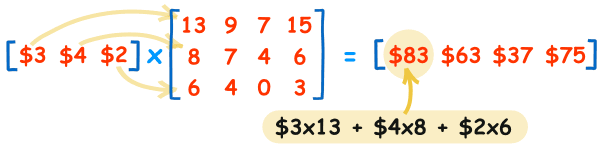（你可以把这些值打进矩阵计算器来看看。）

行与列• 第一个矩阵的列数必须是等于第二个矩阵的行数
• 相乘的结果具有第一个矩阵的 行数 和第二个矩阵的 列数

例子：m×n矩阵与n×p矩阵相乘，n 必须相同，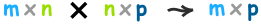乘法的次序

3 × 5 = 5 × 3
（乘法的互换律

AB ≠ BA

例子：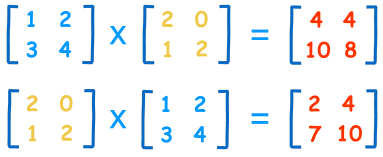单位矩阵

"单位矩阵" 是矩阵领域里的 "1":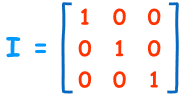3x3 单位矩阵

• 单位矩阵是"方形"的（行与列数目相同），
• 对角线全是1，其他全是0
• 符号为大写字母 I

A × I = A

I × A = A